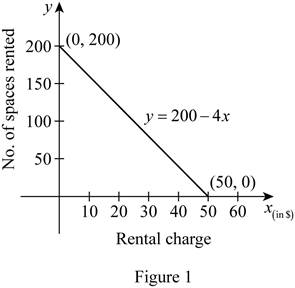# The graph of the linear function y = 200 − 4 x .### Single Variable Calculus: Concepts...

4th Edition
James Stewart
Publisher: Cengage Learning
ISBN: 9781337687805### Single Variable Calculus: Concepts...

4th Edition
James Stewart
Publisher: Cengage Learning
ISBN: 9781337687805

#### Solutions

Chapter 1.2, Problem 12E

(a)

To determine

## To sketch: The graph of the linear function y=200−4x .

Expert Solution

### Explanation of Solution

Let x-axis be represented the rental charge in dollars and the y-axis be represented the number of spaces rented.

Given that the linear function of number of spaces is y=2004x .

Here, the slope of the linear equation is −4 and the y-intercept is 200.

Obtain the value of y for various values of x and draw the graph of y as shown below in Figure 1.From Figure 1, it is observed that the value of x-intercept is 50.

(b)

To determine

### To explain: The meaning of the slope, y-intercept and the x-intercept of the graph obtained in part (a).

Expert Solution

The value of slope represents the rate of change of spaces rented as the rental space changes.

The y-intercept represents the number of occupied space.

The x-intercept represents the rental fee.

### Explanation of Solution

Slope denotes the rate of change of spaces rented as the rental space changes. The negative sign of the slope indicates that the number of spaces rented decreased by 4 as the rental space increases for \$1 each.

The y-intercept is 200, which represents that the number of occupied space when there is no charge for each space.

The x-intercept is 50, which represents the rental fee even there is no space is occupied.

### Have a homework question?

Subscribe to bartleby learn! Ask subject matter experts 30 homework questions each month. Plus, you’ll have access to millions of step-by-step textbook answers!